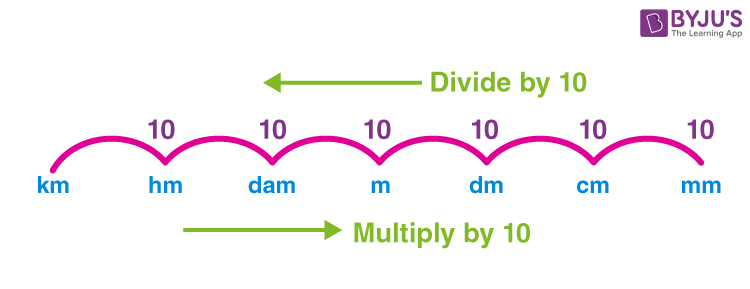# Length Conversion

In mathematics, length is referred to as the distance between two points or the measure of a distance between two endpoints of an object. In other words, length is the measurement of things from end to end. However, it is important to express the length in an understandable format. For this, we use units. For example, the length of a string is 5 metres, the length of a pencil is 15 cm, and so on. Also, it is possible to convert the length from one unit to another. In this article, you will learn the meaning of length conversion, table, and units of length conversion, along with solved examples.

Also, check: Length

## What is Length Conversion?

The method of converting the measures of length from one unit to another is called length conversion. In the metric system, units for length are kilometres (km), metres (m), centimetres (cm), and so on. The conversion of these units from one unit to another is referred to as the conversion of units for length. Thus, we can convert km to m, m to cm, cm to mm and vice versa.

### Length Conversion Chart

The chart for length conversion is given below:## Length Conversion Table

Consider metre as the base unit of length and based on this we can define the conversion factors for length. The below table shows the conversion factors of length from metres (m) to other units of length.

 The basic unit of length (metre) Conversion factor to other metric units Simple length conversion units 1 m 1000 millimetres 1 km = 1000 m   1 m = 100 cm   1 cm = 10 mm   1 hm = 100 m 1 m 100 centimetres 1 m 10 decimetres 1 m 1/10 decametres 1 m 1/100 hectometres 1 m 1/1000 kilometres

The above table shows the metric length conversion values. However, we have other units for length in the Imperial units of measurement. These can be written as given below:

 Imperial measurement of length Conversion factor Conversions to the basic unit of length, i.e. m 1 inch 0.083 foot 0.0254 m 1 foot 12 inches 0.3048 m 1 yard 3 feet 0.9144 m 1 fathom 6 feet 1.829 m 1 rod 5.50 yards 5.029 m 1 furlong 220 yards 201 m 1 mile 1760 yards 1609 m 1 nautical mile 1.151 miles 1852 m

### Length Conversion Calculator

The length conversion calculator helps to convert the measures of length within a fraction of seconds.

Try Length Conversion Calculator here.

### Volume to Length Conversion

Volume to length conversion refers to the conversion of units of volume to the units of length. In some mathematical problems, we may observe that the volume of 3d shaped objects can be expressed in terms of litres (volume units), which often get converted into cm3 or m3 (length units) based on the requirement. In this type of problem, we can use the below conversion formulas.

1 litre = 1000 mL = 103mL = 10−3 m3

1 millilitre = 10−3L = 10−6 m3

1 m3 = 1000 L = 106 mL

1 cm3 = 10−3 L = 1 mL

1 dm3 = 10−3 m3 = 1 L

### Length Conversion Questions with Answers

Question 1: Convert 200 m to km.

Solution:

To convert m to km, divide the number by 1000.

Thus, 200 m = (200/1000) km = 0.2 km

Hence, 200 m = 0.2 km

Question 2: Convert 5.8 m to mm.

Solution:

To convert m to mm, multiply the number by 1000.

So, 5.8 m = 5.8 × 1000 = 5800 mm

Therefore, 5.8 m = 5800 mm

Question 3: Convert 70 decimetres to cm.

Solution:

To convert dm to cm, multiply by 10.

So, 70 dm = 70 × 10 cm = 700 cm

Therefore, 70 dm = 700 cm.

### Length Conversion Worksheet

 How do you convert 4.48 km to cm? How many metres is equivalent to 650 cm? Convert 890 hectometres to dm. 55 km = ____ mm Write 35 cm in hectometres.

## Frequently Asked Questions – FAQs

Q1

### What will be the value of 2.5 km into metres?

We know that 1 km = 1000 metres
2.5 km = 2.5 x 1000 = 2500 metres
Q2

### What will be the value of 3800 metres into km?

We know that 1000 metres = 1 km
3800 metres = 3800/1000 = 3.8 km
Q3

### What will be the value of 90 decimeters into centimetres?

We know that 1 dm = 10 cm
90 dm = 90 x 10 = 900 cm
Q4

### What will be the value of 62 litres into millilitres?

We know that 1 litre = 1000 millilitres
62 litres = 62 x 1000 = 62000 milllilitres
Q5

### What will be the value of 5900 millilitres into litres?

We know that 1000 millilitres = 1 litre
5900 millilitres = 5900/1000 = 5.9 litres